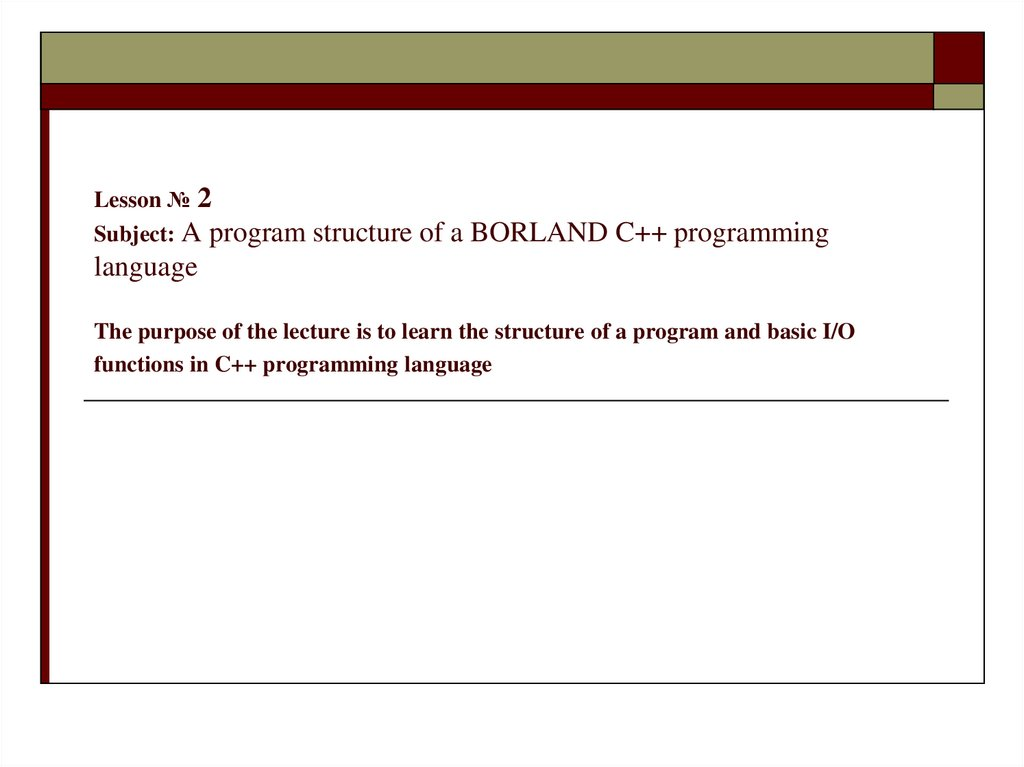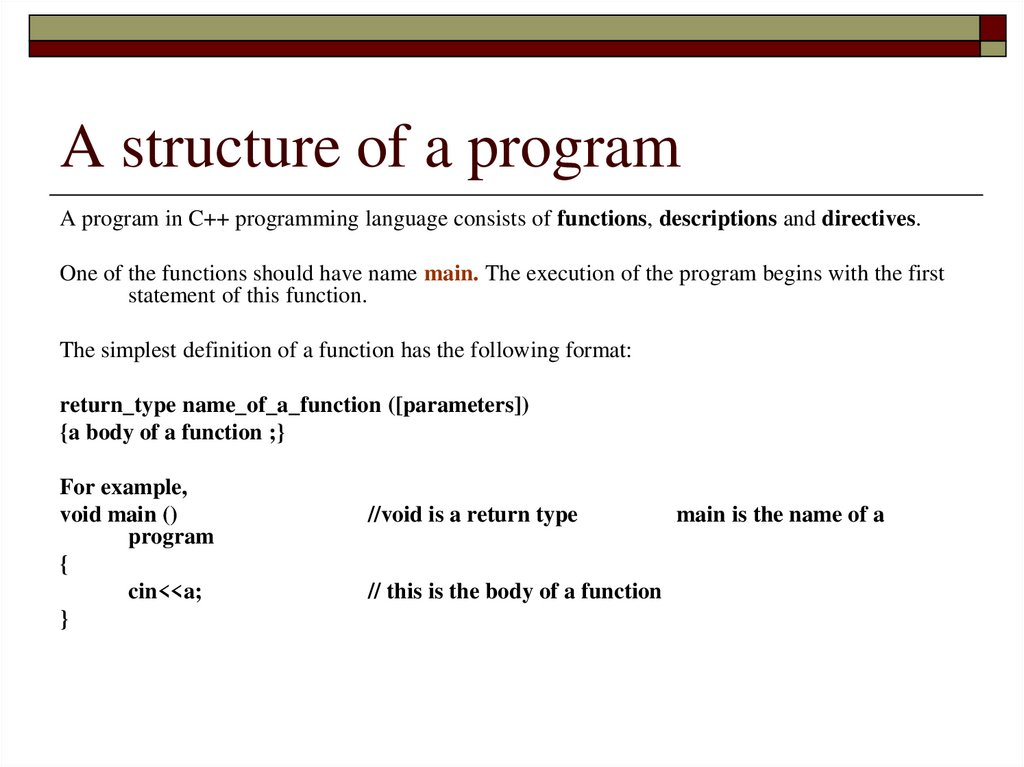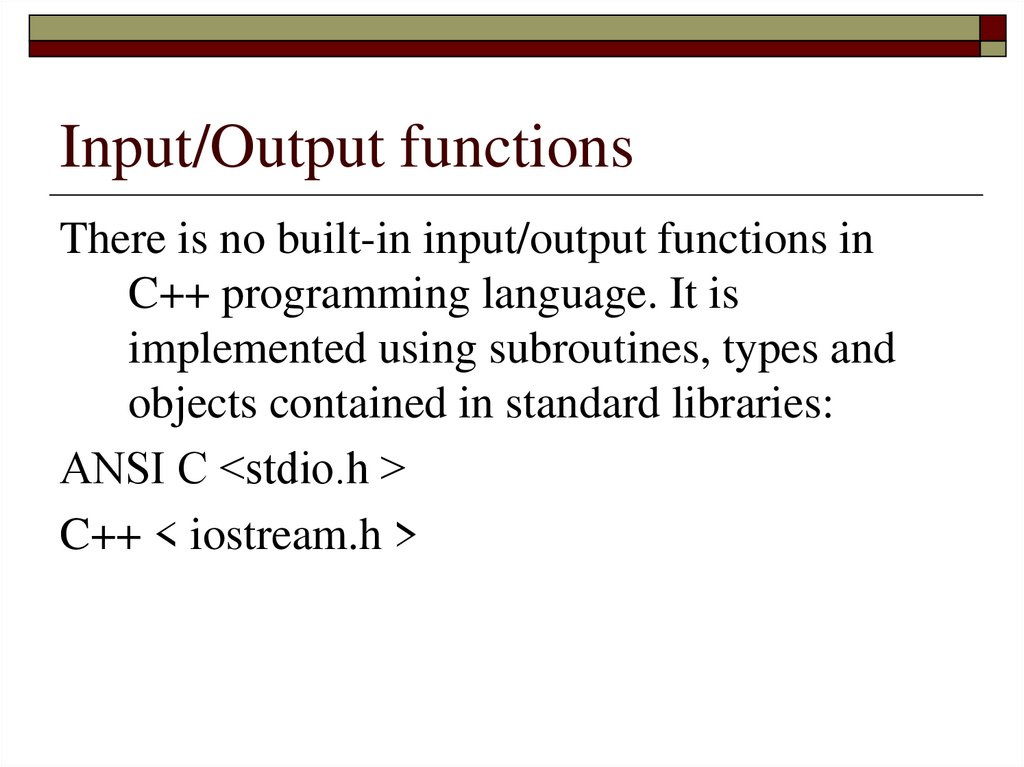# A program structure of a Borland C++

## 1. Lesson № 2 Subject: A program structure of a BORLAND C++ programming language The purpose of the lecture is to learn the

structure of a program and basic I/O
functions in C++ programming language

## 2. A structure of a program

A program in C++ programming language consists of functions, descriptions and directives.
One of the functions should have name main. The execution of the program begins with the first
statement of this function.
The simplest definition of a function has the following format:
return_type name_of_a_function ([parameters])
{a body of a function ;}
For example,
void main ()
program
{
cin<<a;
}
//void is a return type
// this is the body of a function
main is the name of a

## 3. Input/Output functions

There is no built-in input/output functions in
C++ programming language. It is
implemented using subroutines, types and
objects contained in standard libraries:
ANSI С <stdio.h >
C++ < iostream.h >

## 4.

Input/Output functions
Basic I/O functions in a C style:
int scanf (const char* format...)
printf(const char* format...)
//input
//output
They perform formatted input and output of an arbitrary
number of values in accordance with the format string.
The format string contains characters that are copied to the
stream (on the screen) when being output, or are requested
from the stream (from the keyboard) upon input, and
conversion specifications starting with the % character, which
are replaced with specific values upon input and output .

## 5. Example

#include <stdio.h>
int main()
{ int i;
printf(“Enter number\n"); scanf("%d", &i);
printf(“You‘ve entered a number%d, thanks!", i);
}

## 6.

Input/Output functions
And here is what the same program looks like using the
BORLAND C ++ class library <iostream.h>
#include <iostream.h>
int main()
{ int i;
cout << “Enter number\n";
cin >> i;
cout << “You’ve entered a number “<< i << ", thanks!";
}

## 7. Basic data types in BORLAND C++:

Data type
A Size (in bytes)
Value range
char
1
-128..127
unsigned char
1
0..255
short
2
-32768..32767
unsigned short
2
0..65535
long
4
-2147483648..2147483647
unsigned long
4
0..4294967295
int
4
-2147483648..2147483647
unsigned int
4
0..4294967295
float
4
3.4*10-38..3.4*1038
double
8
1.7*10-308..1.7*10308
long double
10
3.4*10-4932..1.1*104932
bool
1
true or false

## 8. Named constants

A named constant is a constant that has a name. A named constant is exactly
like a variable, except that its value is set at compile time (by initializing
it) and CANNOT change at runtime.
Declaring a named constant is a pointer to the compiler to replace (in the entire
text – in a program) this identifier with a constant value .
Constants are added with a keyword const:
const type name_of_a_constant = value;
For example:
const float Pi = 3.14159;
For integer constants, the type can be omitted. The type must be specified for
all other constants.
For example, the next definition
const Pi = 3,14159;
assign a value 3 to the constant Pi.

## 9. Declaration of variables

The declaration of a variable has the form:
type list_of_identifiers;
A list of identifiers may consist of variable identifiers, separated
by commas.
For example: int x1, x2;
Simultaneously with the declaration, some or all of the variables
can be initialized.
For example :
int xl=1, х2=2;
The declaration of variables can be a separate operator or be done
inside of such operators, as, for example, a cycle operator:
for (int i=0; i<10; i++)